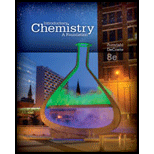# iven each of the following sets of values for three of the gas Variables, calculate the unknown quantity. msp; a . P = 1 .0 34 atm ; V = 21 . 2 mL ; n = 0.00 432 mol ; T = ? K b . P = ? atm ; V = 1 . 73 mL ; n = 0.000 115 mol ; T = 182 K c . P = 1 . 23 mm Hg ; V = ? L ; n = 0. 773 mol ; T = 152 ° C### Introductory Chemistry: A Foundati...

8th Edition
Steven S. Zumdahl + 1 other
Publisher: Cengage Learning
ISBN: 9781285199030

#### Solutions

Chapter
Section### Introductory Chemistry: A Foundati...

8th Edition
Steven S. Zumdahl + 1 other
Publisher: Cengage Learning
ISBN: 9781285199030
Chapter 13, Problem 130AP
Textbook Problem
3 views

## iven each of the following sets of values for three of the gas Variables, calculate the unknown quantity.msp;  a . P =  1 .0 34 atm ; V =  21 . 2 mL ; n =   0.00 432 mol ; T =   ?  K b . P =   ?  atm ; V =  1 . 73 mL ; n =   0.000 115 mol ; T =  182 K c . P =  1 . 23 mm Hg ; V =   ?  L ; n =   0. 773 mol ; T =  152  ° C

Interpretation Introduction

(a)

Interpretation:

To interpret the value of temperature of gas with the help of ideal gas equation.

Concept Introduction:

The pressure, volume and temperature for ideal gas can be calculated with the help of ideal gas. The ideal gas equation is as given below:

PV = nRT

Here R is gas constant that value is 0.0821 L. atm / K. mol.

### Explanation of Solution

Given information:

P = 1.034 atm

V = 21.2 mL = 0.0212 L (1 mL=0.001 L)

n = 0.00432 mole

Plug the values in ideal gas equation;

PV = nRT

1.034 atm × 0.0212 L = 0.00432 mol × 0.0821 L. atm / K. mol × T

T = 1

Interpretation Introduction

(b)

Interpretation:

To interpret the value of pressure of gas with the help of ideal gas equation.

Concept Introduction:

The pressure, volume and temperature for ideal gas can be calculated with the help of ideal gas. The ideal gas equation is as given below:

PV = nRT

Here R is gas constant that value is 0.0821 L. atm / K. mol.

Interpretation Introduction

(c)

Interpretation:

To interpret the value of volume of gas with the help of ideal gas equation.

Concept Introduction:

The pressure, volume and temperature for ideal gas can be calculated with the help of ideal gas. The ideal gas equation is as given below:

PV = nRT

Here R is gas constant that value is 0.0821 L. atm / K. mol.

### Still sussing out bartleby?

Check out a sample textbook solution.

See a sample solution

#### The Solution to Your Study Problems

Bartleby provides explanations to thousands of textbook problems written by our experts, many with advanced degrees!

Get Started

Find more solutions based on key concepts

Understanding Nutrition (MindTap Course List)

The best way to control salt intake is to cut down on processed and fast foods. T F

Nutrition: Concepts and Controversies - Standalone book (MindTap Course List)

6.43 Define the term spin paired.

Chemistry for Engineering Students

What is hereditarianism, and what is the invalid assumption it makes?

Human Heredity: Principles and Issues (MindTap Course List)

Three objects are connected on a table as shown in Figure P5.31. The coefficient of kinetic friction between th...

Physics for Scientists and Engineers, Technology Update (No access codes included)